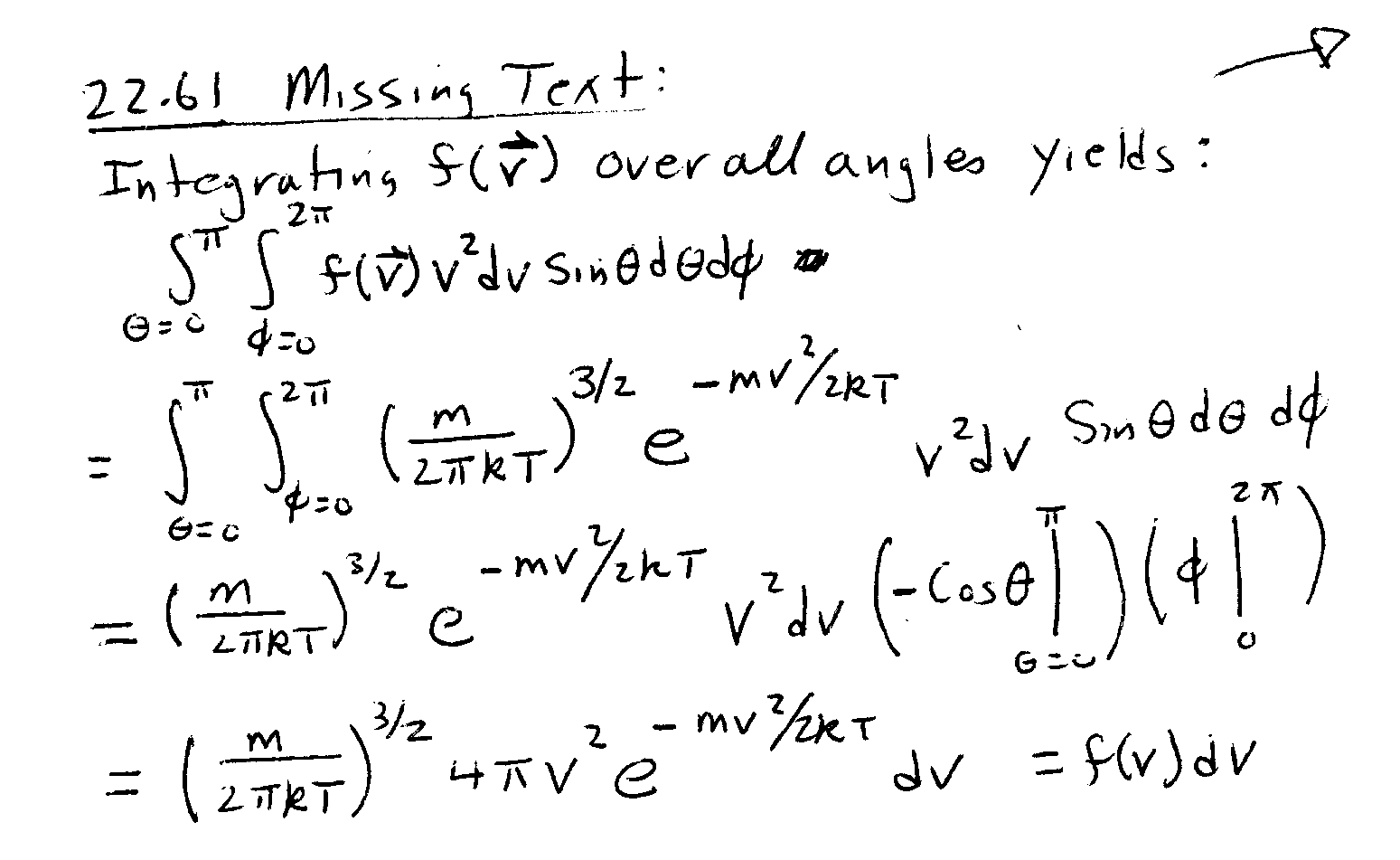### Errata in Solutions Manual

Updated 11/6/00
Page 38
Problem 3.51: Change sign of y-component of acceleration from - to +.
Page 38
Problem 3.51 continued: In the expression for the position vector, the units should be m, not m/s; the sign of the y-component should be -, not +, and the curly brackets should close after the unit vector j, not after the r0
Page 69
Problem 4.99. The 6th line from the end (in the 2nd column) should read: "So if theta is not equal to 0"
Page 89
Problem 5.70 Factor of 2 in denominator of expression for a should be removed.
Page 95
Problem 5.82. In first two answers, g/9 should be g/11. Final answer should be v=11.3 m/s.
Page 108
Problem 6.62 The speed 2.5 m/s is missing from the answer.
Page 145
Problem 8.43. The label on the first part of the solution should be (a) not (i).
Page 210
Problem 11.32. Answer should be 330 N
Page 250
Problem 12.88. 103 should be 102
Page 254
Problem 12.98. Answers should be 14 ° , 50 N · m.
Page 256
Problem 12.104 1.5 should be 1.3
Page 277
Problem 13.114. Argument of inverse tangent should be the square root of M/m not m/M.
Page 291
Problem 14.60 6 should be 3.
Page 294
Problem 14.72: Answer should be 0.056 s
Page 315
Problem 16.42 Answer should be 18 m.
Page 329
Problem 17.22. (m+1/2) should be (2m+1). Maximum value of m should be 5 not 10.
Page 335
Problem 17.53. The figures are out of place. The first figure corresponds to t=1/(4fB).
Page 336
Problem 17.56. 17% should be 4%.
Page 356
Problem 18.67 m = + 0.4 should be m = -0.4.  The sign was dropped in the first line of the solution for m, when the value was substituted for xi.
Page 365
Problem 18.110. 4/7 should be 1/6.
Page 383
Problem 19.90 Answer should be 10 m.
Page 387
Problem 20.34 2.5% should be 8%.
Page 393
Problem 21.16 Answer should be 1.5x10-3K/W.
Page 410
Problem 22.52 1018 cm should be 1012 m, which is about the diameter of the solar system.
Page 412
Problem 22.61: There is some missing text below the figure.Then the numbers 22.62 and 22.63 are missing. The answer to problem 22.62 starts with S0, right below the missing text. The solution to problem 22.63 starts with "Let n = "
Page 413
The numbers 22.64 through 22.67 are missing. Answer to problem 22.64 starts with Qcold. Solution to problem 22.65 starts with "For the diesel..." Answer to problem 22.66 starts with Tf in the second column. Solu tion to problem 22.67 starts with "When heat is added..."
Page 414
Number 22.68 is missing. The answer to problem 22.68 starts with P2 = and includes the table.
Page 424
Problem V.6: 105 should be 104.
Page 440
Problem 24.8: 1016 should be 1015.

Problem number for solution to 24.9 is missing.
Page 484
Problem 26.32. 1.5% should be 0.015%.

Problem 27.46: Answers should be: C = 35 pF; QFree = 53 pC; sigmaouter = 3.4 nC/m2; sigmainner = -6.7 nC/m2; sigmabound,inner = -2.5 nC/m2; sigmabound,outer = 4.8 nC/m2;
Page 506
Problem 27.43. Units of the answer should be F/m
Page 516
Problem 28.30 Answer should be 5x10-6  T.
Page 518
Problem 28.36 (b) and (c) should have 2 sig fig.  in (b), 3 should be 2.6 and in (c) 1 should be 1.3.
Page 562
Problem 30.66. Insert a minus sign in value of B in answer (b): -1.1 nT, and change direction from positive z-direction to negative.
Page 620
Problem 33.20: The phase constant for both E and B should be 0.330 rad.

Problem 33.28 The answer for B should have only 1 significant figure, i.e. 3 not 2.6.
Page 621
Problem 33.34: Answer should be 6.4 micro-N.
Page 649
Problem 34.84: 2nd answer should have 2 significant figures: 13 d.
Page 671
Problem 36.16 The atomic mass number is on the wrong side of the element symbol: 10B not B10
Page 672
Problem 36.18: Answers should be 7.68 MeV and 92.1 MeV.
Page 674
Problem 36.44: Answer should be 10.41 MeV.
Page 676
Problem 36.56: The last nuclide in the reaction chain should be 235U, but the reaction chain is tangential to the point of the question.
Page 678
Problem 36.70: 2nd answer should have 4 significant figures: -28.10 MeV.

Problem 36.72. Answer should have more figures: 3.536x10-11 J/g.
Page 679